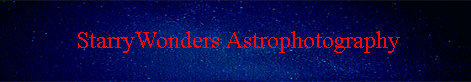CCD "Supercooling"- Should we just chill out?

Introduction: Sure, cooling is a good thing, but how much cooling do we really need to be in that "sweet spot" for amateur, ground-based astrophotography. There are two aspects to this argument that I would like to present separately, one involving the signal to noise ratio (SNR), and the other involving dynamic range.  The discussion involving the SNR is very straightforward but still interesting.  The dynamic range argument introduces a new metric that may take some getting used to.  Both discussions (SNR and dynamic range) are accompanied by interactive graphs and Excel spreadsheets that allow you to explore these topics in greater detail.

I.  How does cooling affect the Signal to Noise ratio?

It is well known that CCD chips generate thermal electrons at a given rate known as the dark current, which is related to chip temperature.  The higher the chip temperature, the higher the dark current.  The dark current, like sky flux or object flux, is a source of noise that must be considered when calculating the signal to noise ratio. Below I will modify the standard signal to noise equation discussed earlier to account for the contribution of dark noise.  In the resultant interactive graphs (requiring Quicktime 7 or later), you will see that the effect of the dark noise contribution becomes minimal when the chip is cooled to the range of -15 to -20 C, assuming that your conditions are typical of most ground based, amateur imagers.  If you want to skip the math, just drop down to section B below (Interactive Graphs), but you will be missing a lot of fun <g>.

A) Derivation:

1.  The signal to noise ratio per pixel for a single sub is usually expressed as follows (ignoring the contribution from dark noise):
SNR = (Obj)*tsub / sqrt[(Sky+Obj)*tsub + R2]

where SNR = signal to noise ratio per pixel; Obj = object flux in electrons/minute/pixel; Sky = sky flux in electrons/minute/pixel; tsub = subexposure time in minutes; R = read noise in e RMS.

2.  For the purpose of this discussion, we want to include dark noise in the analysis, so let's represent the dark current as D, in electrons/minute/pixel (for instance).  The equation simply becomes:
SNR = (Obj)*tsub / sqrt[(Sky+Obj+D)*tsub + R2]

3.  The dark current (D) doubles with a constant delta increase in temperature, which we will call the doubling temperature (Td).  For most CCD chips this is around a delta of 6 degrees centigrade.  So if the dark current is 30 electrons per minute at 0 degrees, it would double to 60 electrons per minute at 6 degrees, 120 electrons per minute at 12 degrees, etc.  Conversely, if the CCD temperature decreases by a delta of 6 degrees C, the dark current D would be cut in half.  This kind of relationship is analogous to exponential growth of bacteria in an environment that has unlimited space and nutrients, or from an oncologist's point of view, exponential growth of cancer cells.  (In reality, neither growth of bacteria nor cancer cells follows exponential growth forever, since proliferation is limited by nutrients, oxygen delivery, and space.)  The mathematical way of expressing this type of exponential relationship is by saying that the rate of change of D (dD) with respect to the change in chip temperature (dT) is proportional to D:

dD/dT  = kD, where k is a rate constant, D is the dark current in e/minutes/pixel, and T is CCD temperature in centrigrade.

4.  Rearranging this we get dD/D = kdT.  Integrating this we obtain:

Equation 4:  ln (D) = kT + C, where C is the integration constant.

5.  At T = T0, D = D0.  (these are often stated in the manufacturer's specs for a given CCD chip.  For instance, the dark current might be stated as 30 electrons/minute (D0) at 0 degrees C (T0).

6.  The constant, C, is then:

ln (D0) = k(T0) + C;  C = ln (D0) -k(T0)

7.  Substituting this into equation 4:

ln (D) = kT + ln (D0) - k(T0).  Rearranging we get:

Equation 7:  ln (D/D0) = k(T-T0)

8.  Solving for k, we get:

Equation 8:  k = [ln (D/D0)] / (T-T0)

8.  We can determine k for a given CCD chip based upon the doubling temperature (Td), again a characteristic of the chip.  For the KAI 11000 Kodak chip, this is approximately 6.3 degrees C.  So for every 6.3 degree C increase, the dark current doubles.  Thus:

k = ln (2/1) / 6.3 = 0.693/6.3 = 0.11

More generally, however, we can simply say that:

Equation 8:  k = 0.693/Td

9.  From equation 7, we can solve for D, the dark current:

ln (D/D0) = k(T-T0).  Substituting k with equation 8, we get:

ln (D/D0) = (0.693/Td)(T-T0) = 0.693*[(T-T0)/Td] where Td is the doubling temperature.  Thus:

D/D0 = e0.693*[(T-T0)/Td]

Equation 9:  D = D0*e0.693*[(T-T0)/Td]

10.  Now we are ready to add this term to our SNR equation:
SNR = (Obj)*tsub / sqrt[(Sky+Obj+D0*e0.693*[(T-T0)/Td])*tsub + R2]

B) Interactive Graphs:

Now onto the fun!   Equation 10 allows us to study the effect of varying the CCD temp on the SNR under a variety of conditions.  For this purpose, I used a wonderful graphing program entitled "Graph" from the following website (http://www.padowan.dk).  Explore each of the graphs below in order to get a feel for the relationship between CCD cooling and SNR.  Please note the following caveats:

Caveat 1.  This analysis is using the average dark current, which is representative of the vast majority of pixels on a given chip.  I have purposefully ignored the contribution of the very hottest pixels (which have a very high dark current) to the overall noise.  The justification for this is simple- As will be discussed in Topic II (Dynamic Range), the number of hot pixels in a given CCD chip is a very small fraction of the total number of pixels, and their impact on the SNR of the final image is negligible.

Caveat 2.  I have ignored the contribution of dark signal non-uniformity in this analysis (of which hot pixels represent a special case).  Again, the reason for this will become clear in the section II, but the bottom line is that the variation of dark current for the majority of pixels in a given CCD chip is surprisingly low.  It is true that some pixels exhibit more variability than others (i.e., the warm and hot pixels), but because they represent only a few percent of the total pixel population, they can easily be ignored for the purpose of "pretty picture" work.  This is especially true if your subs are dithered (a good practice!) followed by combining using a Sigma Reject algorithm.

Caveat 3.  None of the graphs below incorporates the additional noise that would be introduced by calibration, especially as it relates to dark frame subtraction.  The reason for this is simple- even at high CCD temperatures, the added influence of dark noise introduced through the act of subtracting a well-constructed master dark frame is very minor compared to other sources of noise, and it does not change the shapes of the curves nor the conclusions of this analysis.

Explore the various scenarios below in order to learn how the SNR varies with CCD temperature, and the dependency of this relationship on various parameters like Sky flux, Read noise, and D0.  You may be surprised...

Example 2:  Vary the Read noise.  Same parameters as in #1, but with a fixed sky flux of 20 e/min (typical of my site with the STL11K and a 6nm Ha filter)Click on the this link to open a graph of SNR (y axis) as a function of CCD temp (x axis).  The slider will vary the read noise from 2 to 15 e RMS as it moves from left to right (incremental steps of 0.5 e RMS).  Notice that over a wide range of read noise values, the shape of the curve does not change much, and the added value of extreme cooling is present but minimal.  I have tested this graph for both low sky flux and low dark current conditions and find the same general relationship between CCD temperature and SNR as a function of changing read noise (graph not shown).

Example 3:  Vary the baseline D0 value (i.e., the dark current specification of the chip at T0).  Even though D0 is a characteristic of the chip as opposed to a variable under our control, it is instructive to see how this parameter affects the SNR.  This graph uses the same parameters as in #1, but with a fixed sky flux of 20 e/min (typical of my site with the STL11K and a 6nm Ha filter).  Click on the this link to open a graph of SNR (y axis) as a function of CCD temp (x axis)The slider will vary the D0 value from 1 to 50 e/min as it moves from left to right (incremental steps of 1 e/min)Notice that a low baseline dark current (slider all the way to the left), as might be seen with the KAF 16803 for instance (D0 of 10 e/min at at T0 = 0 degrees C) will preserve the SNR at temperatures that most would have considered too high for imaging!  Conversely, the major impact of a high baseline D0 value is in the high temperature range- a noisy chip with a high dark baseline dark current would be a bad choice for uncooled DSLR imaging!  But once your chip temperature gets to about -15 to -20 C, the SNR is optimized even with a high dark current chip like the KAI 11000 (D0 of 30 e/minutes at 0 degree C).  Again, this assumes that one is imaging on earth, through the atmosphere, where sky noise becomes the dominant source of noise.

Bottom Line:  Each of these three examples shows that you gain very little signal to noise by cooling much below -20 C under most circumstances relevant to amateur astrophotographers.  I consider this to be a general "rule" because this observation is quite insensitive to the initial parameters listed above and is largely driven by the fact that sky noise is the limiting factor for most ground-based imaging.  You can cool the chip all you want, but you can't get rid of sky noise.  However, if you are designing a camera that will operate in deep space, where sky glow is not an issue, then it is certainly desirable to push CCD cooling as far as possible.  The final point made by the graph in example 3 is especially relevant to more recent chips like the KAF 16803.  Such chips have low dark current and can produce an excellent, sky limited SNR at CCD temperatures formerly thought to be unacceptably warm.  There is simply no need to push these newer chips very hard in order to produce wonderful, clean looking images with excellent signal to noise.  Thus, determining how much to cool a CCD chip depends on a number of factors, not the least of which are the quality of the imaging site (sky flux) and the intrinsic chip characteristics (D0).  For most of us, these factors impose an upper limit on the SNR and minimize the effects of CCD cooling on image quality, once you reach the range of -15 to -20 C.

We are almost done this section!  It is easy to calculate the maximum possible SNR for a given set of initial conditions, under the idealized situation in which the dark current is zero.  This equation is already shown above in point #1.  The ratio of actual SNR at a given CCD temperature to the maximum possible SNR is then obtained by dividing equation 10 by equation 1.  I have made an Excel Spreadsheet that allows you to input initial conditions, state your desired CCD temperature, and then determine how this affects your ability to achieve the maximum possible SNR.  You will see that for most real world conditions of amateur astrophotographers, being in a range of -15 to -20 degrees C allows us to achieve a significant fraction of the maximum possible SNR.  There is very little to be gained by extreme cooling of the CCD chip beyond -20C under most of the circumstances that we face.  You can see, however, that if you wish to place your CCD outside of the earth's atmosphere (sky flux of 0) and are imaging a faint object (object flux of 1), it is advantageous to have a very cold chip.  You can also appreciate how the SNR is impacted at fairly warm temperatures (e.g., 25 degrees C), especially at a dark site and with a chip that has a high baseline dark current.  You are welcome to experiment with this spreadsheet to get a feel for the numbers.

II.  How does cooling affect the dynamic range?

A) Background:

The above considerations suggest that from the standpoint of signal to noise, one reaches a point of diminishing returns once the CCD chip is cooled to about -20 C or so (the exceptions have been mentioned above but are not very relevant to most amateur astrophotographers who image at relatively light polluted sites).  However, there is another effect of dark current that we need to consider, as it relates to the "signal" that it generates from thermal electrons.  These non-specific electrons generated by the dark current take up valuable space within the pixel well, leaving less room for object-specific signal.  For instance, if a pixel has a well depth of 50,000 electrons, but a very high dark current that fills the well with 40,000 electrons over a 20 minute sub, then you can only accomodate 10,000 electrons for real signal.  For a fairly bright object, such a pixel will easily be clipped in the highlights, and you have lost valuable information.  So we can ask- how much of a problem is this, and how does the CCD temperature affect this?

This definition of dynamic range is fine for characterizing what a chip can do under the ideal conditions where no other sources of signal or noise exist.  However, that is not reality.  In the real world, our ability to accumulate object specific signal (and therefore take advantage of the dynamic range) is impacted by 1) the degree to which undesirable "other" electrons accumulate in the well (such as those introduced by thermal electrons and sky flux), and 2) the degree to which sky flux and dark current contribute to the total noise.  For the analysis that follows, I would like to define another way of looking at dynamic range, which I will call the "functional dynamic range," that captures all of the factors that influence our ability to effectively use a given CCD chip's full well capacity:

Equation 12.  "Functional Dynamic Range" = [FW - sky*tsub - D*tsub] / SQRT[sky*tsub + D*tsub +R^2], where FW is the full well capacity in electrons, sky is the sky flux in electrons/minute, D is the dark current in electrons/min, and R is the read noise in e RMS.

Notice what this new definition does.  It recognizes the negative impact of sky flux and thermal electrons on the full well capacity (those electrons are subtracted out of the FW in the numerator), and it also recognizes that for an actual image, we don't just care about read noise, but we need to account for all of the noise components that dictate the ability of the chip to render the very faintest and brightest signals.  The functional dynamic range is the metric that I will use to determine how dark current affects the dynamic range of an image.

From equation 9, we know that D = D0*e0.693*[(T-T0)/Td] .  Substituting this into equation 12, we get:

Equation 13.  Functional Dynamic Range = [FW - tsub*(sky + D0*e0.693*[(T-T0)/Td])] / SQRT[tsub*(sky + D0*e0.693*[(T-T0)/Td])+R^2]

We will shortly use this equation in few interactive graphs to illustrate the effect of CCD temperature on dynamic range.

B) One picture is worth a thousand words (or equations)...

Before we proceed further, I need to convince you that we can safely ignore dark current outliers for much of the analysis of dynamic range.  Here is a bias calibrated histogram of a dark frame from my STL11000 taken at -20C for 20 minutes: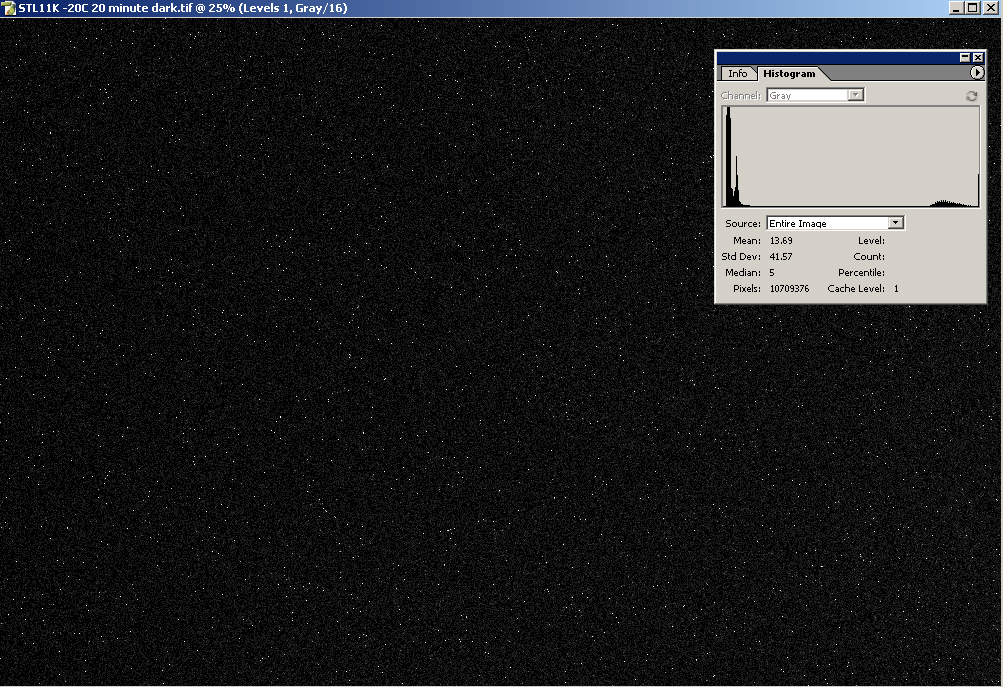As shown below, the histogram can be divided into distinct regions occupied by pixels with varying amounts of dark current.  Region A is comprised of low intensity pixels that represent the most common pixel species (96%).  Pixels in this location have most of their full well available to them.  In fact, if you want to be even more accurate, Region A is comprised of two populations of low dark current pixels, but I didn't see the need to split it into Region A1 and A2 for the purpose of this analysis.  Regions B, C, and D represent pixels with varying degrees of higher dark current, but it is important to note that collectively they comprise only 4 percent of the total.  Within this group is a population of saturated pixels (Region D) or near saturated pixels (Region C). From a dynamic range standpoint, these pixels are essentially worthless to us, but thankfully they comprise only 2.6% of the population.  Region B is comprised of warm pixels that have a non-uniform dark current.  Although they have the potential for capturing real signal, they only constitute 1.4% of the total.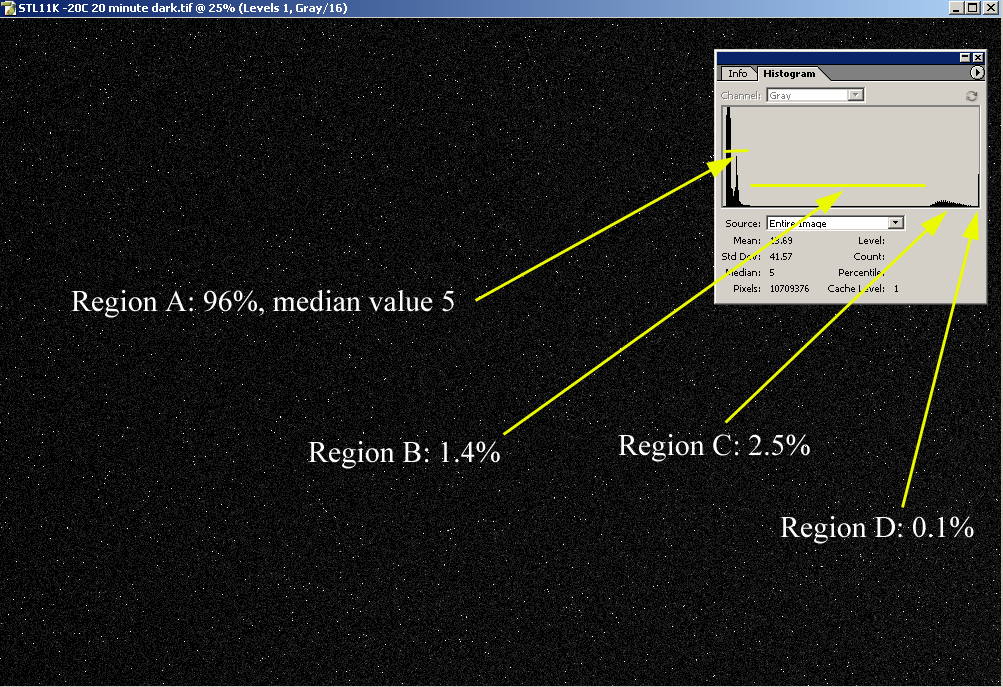Here's what happens when you increase the CCD temperature by 5 degrees.  The figure below shows a bias calibrated histogram of a dark frame from my STL11000 taken at -15C for 20 minutes: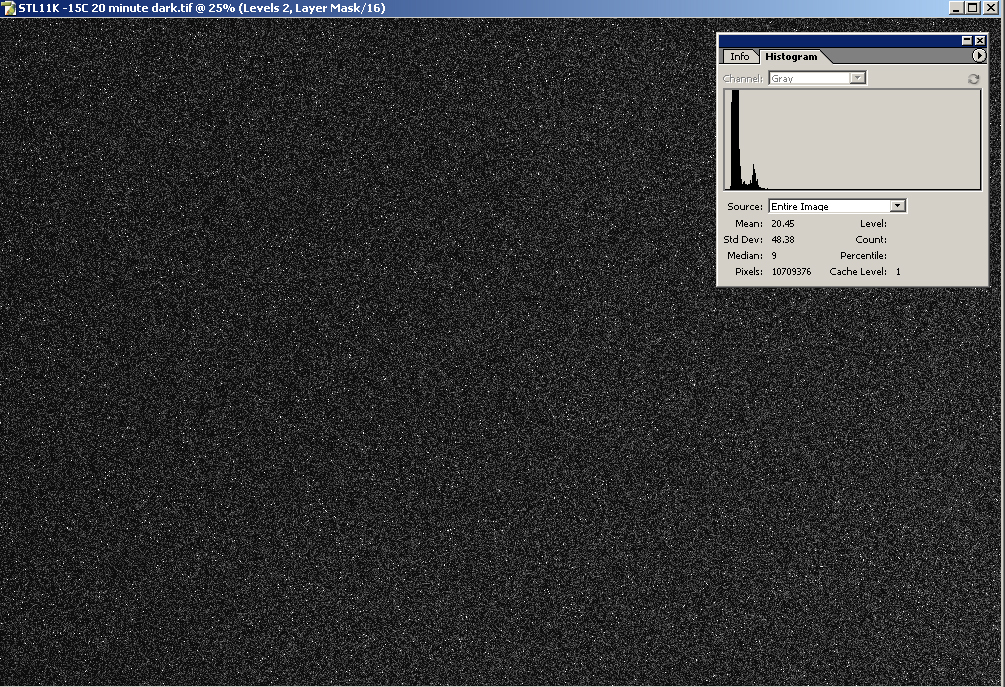Notice what has happened.  Regions C and D seen in the -20 degree histogram have now collapsed into one saturated peak of clipped pixels that is difficult to appreciate but is present as a thin line on the far right, comprising 3.9 % of the population (Region C below).  Region B is again comprised of pixels with a non-uniform dark current, many of which have the potential to accumulate real signal, but they only comprise 1.1% of the total.  The small fraction of pixels in regions B and C are part of the dark signal non-uniformity (DSNU), which is fixed pattern noise characteristic of a given CCD chip.  Because they are always fixed in location, the effects of these higher noise pixels on the signal to noise ratio can easily be eliminated by dithering your images, followed by combining using a Sigma Reject algorithm.  For this reason, they are of minor consequence to the signal to noise of the final image, and they will be ignored for the purpose of this analysis.  Again we see that the most functionally capable population of pixels, Region A, represents 95% of the total.  Visually you can appreciate that Region A has eaten into the dynamic range a bit, since the median has shifted over to 9 (the median was around 5 at -20C).  It is this shift that we will be quantifying in our analysis of the relationship between dynamic range and CCD temperature.  In other words, the distribution of pixel intensities in these dark histograms make it reasonable to ignore the small population of warm or hot pixels, and to focus our attention on the bulk of the pixels on the left end of the histogram, which can be approximated by the average dark current equation (Equation 9).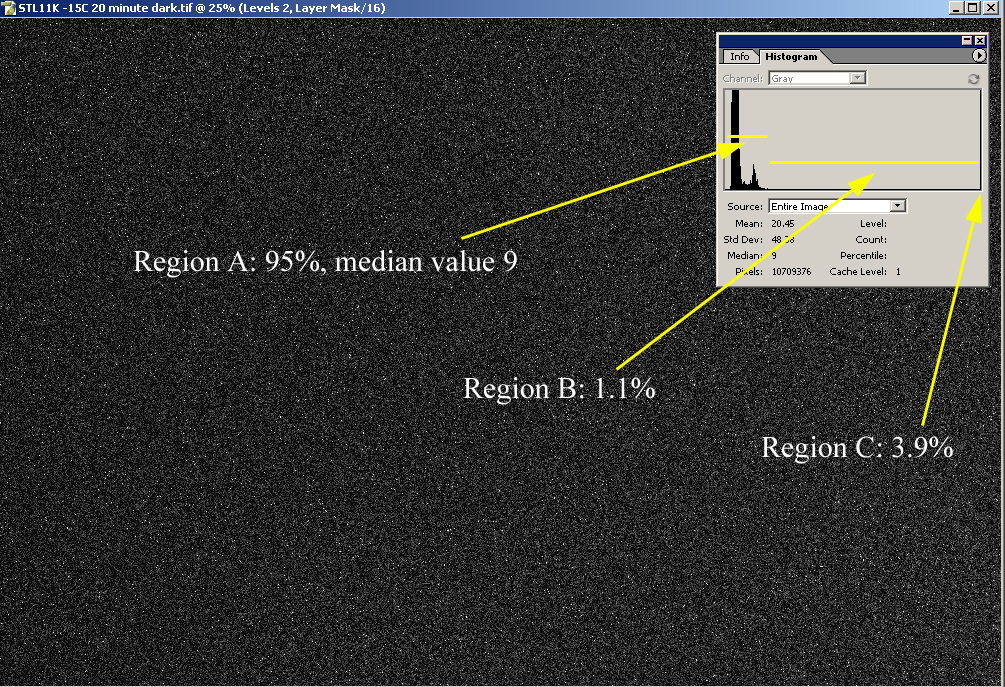C) Interactive Graphs:

Equation 13 allows us to graph the functional dynamic range versus CCD temperature under a variety of conditions.  As before, here are a few scenarios:

Example 1:  Vary the Sky flux.  Parameters as follows:  R = 8 e RMS; D0 = 30 e/minute/pixel at T0 = 0 degrees C; Td = 6.3 degrees C (D0, T0, and Td characteristic of the KAI 11000 chip).  Click on the this link to open a graph of Functional Dynamic Range (y axis) versus CCD temp (x axis).  The slider will vary the sky flux from 0 (e.g., the Hubble) to 500 (a light polluted imaging site) as it moves from left to right (incremental steps of 10 e/min).  Notice how the functional dynamic range is affected by CCD temperature in the range of -15 to -20 C at various sky flux values.  When the slider is all the way to the left (sky flux = 0; deep space), we see that the achievable dynamic range is high, and that the benefit of additional cooling increases until the CCD temperature reaches the range of -40 to -50 degrees C, at which point it begins to plateau.  However, even with relatively small increments in sky flux, the functional dynamic range begins to drop very quickly, associated with a flattening of the curve, such that very little gain in the functional dynamic range is observed after achieving CCD temperatures in the range of -15 to -20 degrees.  Again, this can be predicted from Equation 13- as the sky flux grows larger, it dominates both the numerator and the dominator, thereby dimishing the influence of dark signal (and read noise) on dynamic range.

Example 2:  Vary the Read NoiseSame parameters as in #1, but with a fixed sky flux of 20 e/min (typical of my site with the STL11K and a 6nm Ha filter).  Click on the this link to open a graph of Functional Dynamic Range (y axis) versus CCD temp (x axis).  The slider will vary the read noise from 2 to 15 as it moves from left to right (incremental steps of 0.5 e RMS).  The influence of read noise on the shape of the curve is minimal in the CCD temperature range of -15 to -20 C under these conditions.

Example 3:  Vary the baseline D0 value (i.e., the dark current specification of the chip at T0).  Even though D0 is a characteristic of the chip as opposed to a variable under our control, it is instructive to see how this parameter affects the functional dynamic range.  This graph uses the same parameters as in #1, but with a fixed sky flux of 20 e/min (typical of my site with the STL11K and a 6nm Ha filter).  Click on the this link to open a graph of Functional Dynamic Range (y axis) versus CCD temp (x axis)The slider will vary the D0 value from 1 to 50 e/min as it moves from left to right (incremental steps of 1 e/min).  Notice the profound effect that D0 has on the functional dynamic range at warm CCD temperatures, but how immune the curve is to dark current once the chip has been cooled to the -15 to -20 C range.  In fact, even at a chip temperature of 0 degrees C (which most of us would consider too warm for imaging), a very low D0 value can support an excellent level of functional dynamic range!  A stated above, the KAF 16803 is typical of such a chip (with a D0 of 10 e/min at T0 = 0 degrees C).

Bottom line:  As in the discussion involving the SNR, the influence of CCD cooling on dynamic range is largely determined by imaging site (sky flux) and chip characteristics (D0).  CCD cooling beyond -15 to -20 C produces minimal gains in the functional dynamic range for most imaging sites, because the sky flux becomes the limiting factor in this situation (graph in example 1).  As CCD chips are manufactured with better dark current characteristics (D0), the need for extreme cooling becomes even less (graph in example 3).  This is interesting, because when the KAF 16803 chip was introduced into amateur astrophotography, it seemed that the emphasis was on achieving even greater cooling, when in fact these chips would be perfectly happy with less.  Nonetheless, it is important to note that the functional dynamic range is optimized at very cold CCD temperatures under conditions of low sky flux, such as one would find in deep space.

If you have made it this far, you are either very committed, very crazy, or both <g>.  It is easy to calculate the maximum possible functional dynamic range for a given set of initial conditions, under the idealized situation in which the dark current is zero.  This equation would simply be equation 13, but without the dark current term:

Equation 14.  Maximum Functional Dynamic Range = [FW - (tsub*sky)] / SQRT[(tsub*sky)+R^2]

The ratio of actual functional dynamic range at a given CCD temperature to the maximum possible functional dynamic range is then obtained by dividing equation 13 by equation 14.  I have made an Excel Spreadsheet that allows you to input initial conditions, state your desired CCD temperature, and then determine how this affects your ability to achieve the maximum possible functional dynamic range.  You will see that for most real world conditions of amateur astrophotographers, being in a range of -15 to -20 degrees C allows us to achieve a significant fraction of the maximum dynamic range.  Notice, however, what happens when you use a sky flux value of 0 (deep space).  Here is where extreme CCD cooling really shines, but hopefully this analysis has convinced you that this is the exception rather than the rule.  You are welcome to experiment with this spreadsheet to get a feel for the numbers.

III.  Conclusions

I hope that you've had fun with this analysis.  None of this is intended to inhibit research and development of better cooling systems by manufacturers of amateur CCD cameras.  The marketplace is an "arms race," and everyone is trying to compete with each other to make the best products possible.  This is a good thing for consumers.  But sometimes an idea (like cooling) takes on a life of its own, such that we automatically assume that we need the latest and greatest cooling to be happy in this great hobby of ours.  We sometimes place more emphasis on the technical characteristics of a camera, characteristics that have an over-inflated impact on our images, and not enough emphasis on our own skills as imagers.  To be sure, if I wanted to build a camera to function on board Cassini, you bet that I would want to cool it down as much as possible.  And if I lived in a very warm climate, I would want to be confident that my camera was able to cool to the range of -20 degrees C without too much effort.  But given our skies, and especially given the new revolution in low dark current CCD chips, I contend that ground-based amateurs generally don't need cameras that can cool to -30 C to take great images.  For the most part, we don't even need cameras that can cool to -25 C.  And by imposing more aggressive cooling on these chips, it is possible that we are increasing the likelihood of experiencing other side effects such as residual bulk image (RBI), although that is a topic for another day.

If you enjoyed this analysis, I also recommend that you check John Smith's cooling analysis, which is very informative.

Steve